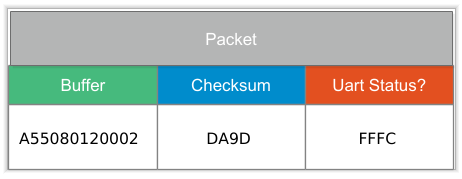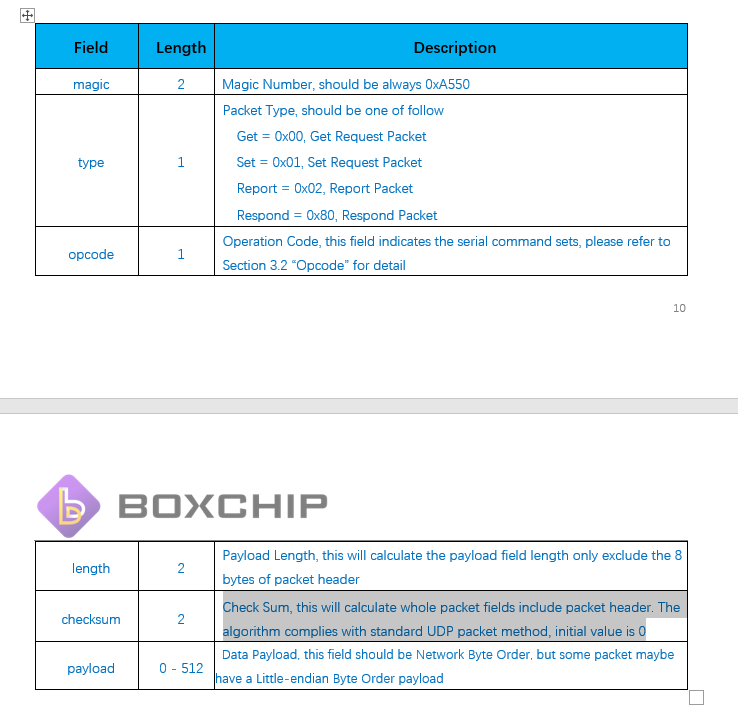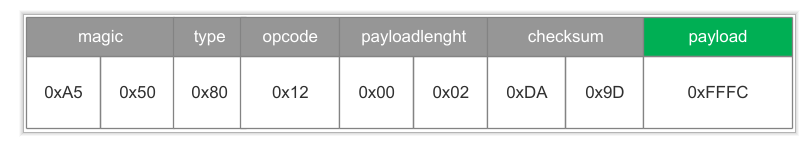# Android QuestionUDP Checksum

#### Philip Prins

##### Active Member
Longtime User
Hello ,

I need to calculate the checksum according to this:
Checksum computation. The method used to compute the checksum is defined in RFC 768: Checksum is the 16-bit one's complement of the one's complement sum of a pseudo header of information from the IP header, the UDP header, and the data, padded with zero octets at the end (if necessary) to make a multiple of two octets.

I have this code:
C++:
``````uint16_t Sys_Checksum(uint16_t init, uint8_t* buff, int32_t len)
{
uint32_t i = 0;
uint32_t checksum = init;

while(len >= 2)
{
checksum += ((buff[i] << 8) & 0xff00) | buff[i + 1];
i += 2;
len -= 2;
}

if(len)
{
checksum += (buff[i] << 8) & 0xff00;
}

while(checksum >> 16)
{
checksum = (checksum >> 16) + (checksum & 0xffff);
}

return (uint16_t)(~checksum);
}``````

How can i do this in B4A?

Regards,
Philip

•emexes

#### Erel

##### B4X founder
Staff member
Longtime User
Do you have a Java implementation?

Is there any example of the input and the expected output?

#### Philip Prins

##### Active Member
Longtime User
Do you have a Java implementation?

Is there any example of the input and the expected output?
Hello Erel,

RX: A55080120002DA9DFFFC
CheckSum DA9D

I hope this helps

#### Erel

##### B4X founder
Staff member
Longtime User
Do you have a Java implementation?

#### Philip Prins

##### Active Member
Longtime User
Do you have a Java implementation?
No only the C code
This was in the documentation:
Check Sum Algorithm
We employ the standard UDP verify algorithm for packet, here is a source code in C language, please set “init” to 0 or the previous calculate value.
Check Sum, this will calculate whole packet fields include packet header. The algorithm complies with standard UDP packet method, initial value is 0

#### Erel

##### B4X founder
Staff member
Longtime User
After some reading, this checksum is used internally by the OS. You will not receive corrupted packets.

#### Philip Prins

##### Active Member
Longtime User
After some reading, this checksum is used internally by the OS. You will not receive corrupted packets.
Good morning Erel,

It is serial communication over a native serial port.
They mention UDP as reference.

When I send packets using the UART library i have to add the checksum otherwise i get an error response.

#### Erel

##### B4X founder
Staff member
Longtime User
You can implement it using the Bit type, however it will be simpler to convert Java code as the types will be the same.

#### Philip Prins

##### Active Member
Longtime User
I tried using different CRC16 examples i found on the forum but got stuck .
Do you have an example how to do this?

#### Philip Prins

##### Active Member
Longtime User
Yes i tried several of them without success.
My knowledge is to limited to determine the difference between the C example and B4A examples.

#### MicroDrie

##### Well-Known Member
It looks to me that:
1. The CRC calculation starts with a secret offset value “uint16_t init”. Without this parameter no CRC calculation can be done.
2. The second variable I suspect is buffer string;
3. The third parameter is the number of bytes for the CRC calculation.
I think reporting a reference to UDP is just too short-sighted. I understand from your story that you are going to send a buffer serially. You start to calculate the checksum over the serial buffer contents.

The calculation of the checksum of a UDP packet contains also the source and destination address from and to where the buffer contents is send to. Serial transmission is a point to point connection with out those source and destination addresses.

Complicated factor is that you RX (received) example contains also the given checksum, but not add the end of the packet. In the second half of the 80 I did some serial port programming. So I expect that a packet is build as follows:
1. The 6 bytes content of the buffer where over the checksum is calculated;
2. The 2 bytes calculated CRC of the buffer;
3. The last 2 bytes, can be anything.
Most likely is this the situation:
RX: A55080120002DA9DFFFC
CheckSum DA9DI need the initial start value of the CRC calculation to check for a correct conversion from C++ to Java.

Last edited:

#### Philip Prins

##### Active Member
Longtime User
It looks to me that:
1. The CRC calculation starts with a secret offset value “uint16_t init”. Without this parameter no CRC calculation can be done.
2. The second variable I suspect is buffer string;
3. The third parameter is the number of bytes for the CRC calculation.
I think reporting a reference to UDP is just too short-sighted. I understand from your story that you are going to send a buffer serially. You start to calculate the checksum over the serial buffer contents.

The calculation of the checksum of a UDP packet contains also the source and destination address from and to where the buffer contents is send to. Serial transmission is a point to point connection with out those source and destination addresses.

Complicated factor is that you RX (received) example contains also the given checksum, but not add the end of the packet. In the second half of the 80 I did some serial port programming. So I expect that a packet is build as follows:
1. The 6 bytes content of the buffer where over the checksum is calculated;
2. The 2 bytes calculated CRC of the buffer;
3. The last 2 bytes, can be anything.
Most likely is this the situation:

View attachment 94815
I need the initial start value of the CRC calculation to check for a correct conversion from C++ to Java.

Hello ,

According to the documentation the initial value is 0.

Check Sum, this will calculate whole packet fields include packet header. The algorithm complies with standard UDP packet method, initial value is 0#### MicroDrie

##### Well-Known Member
That information can be reduced to the following picture:So the CRC is only calculated over the green colored payload whereby the first 8 bytes are ignored. I'm have it very busy now, but I change my test CRC program.

•Philip Prins

#### MicroDrie

##### Well-Known Member
I run into a challenge in the conversion from C ++ to Java with this operation:
B4X:
``while(checksum >> 16) {}``

This should be write out in Java as:
B4X:
``while(checksum >>> 16) {}``

This gives the following error:
javac 1.8.0_241
src\b4j\example\calccrc.java:183: error: incompatible types: int cannot be converted to boolean
while(crc >>> 16){}
^
1 error

Another challenge lies in the fact that the addition of two bytes leads to a checksum value of 0xDA9D.

Another challenge lies in the fact that adding the two bytes (0xFF and 0xFC) would result in a checksum value of 0xDA9D. With reference to UDP checksum, including the pseudo header bytes 0xA5, 0x50, 0x80,0x12, 0x00, 0x02 would therefore be more obvious or the payload buffer has more characters.

#### OliverA

##### Expert
Longtime User
while(checksum >>> 16) {}
Write
B4X:
``while((checksum >>> 16) > 0)``

#### MicroDrie

##### Well-Known Member
I converted the C++ program with B4J and the JavaObject (Versie 2.06) library
B4X:
``````#Region Project Attributes
#MainFormWidth: 600
#MainFormHeight: 600
#End Region

Sub Process_Globals
Private fx As JFX
Private MainForm As Form

Private CRC As CalcCRC
End Sub

Sub AppStart (Form1 As Form, Args() As String)
MainForm = Form1
'    MainForm.Show
CRC.Initialize
CRC.CalculateCRC(0,"FFFC",2)
'    CRC.CalculateCRC(0,"9801331b980e5e4b",16)
'    CRC.CalculateCRC(0,"9801331b980e5e4b0011000a",8)
ExitApplication
End Sub

'Return true to allow the default exceptions handler to handle the uncaught exception.
Sub Application_Error (Error As Exception, StackTrace As String) As Boolean
Return True
End Sub``````
And create this class:
B4X:
``````Sub Class_Globals
Private NativeMe As JavaObject
End Sub

'Initializes the object. You can add parameters to this method if needed.
Public Sub Initialize
NativeMe = Me
End Sub

#IF JAVA
/*
* The converted algorithm is simple, using a 32 bit accumulator (checksum),
* we add sequential 16 bit words to it, and at the end, fold back all
* the carry bits from the top 16 bits into the lower 16 bits.
*/

/*
* uint16_t Sys_Checksum(uint16_t init, uint8_t* buff, int32_t len)
*/

public int CalculateCRC(int init, String buff, int len)
{
/* uint32_t i = 0; */

int words = len;
int        checksum;

/* We need a counter */
int        i = 0;
int            temp;

/* uint32_t checksum = init; */
checksum = init;

/* variables for debuging */
String  s;
BA.Log(buff);

/* while(len >= 2) */
while(len >= 2){

s = buff.substring(i,i+2);
BA.Log(s.toUpperCase());

/* checksum += ((buff[i] << 8) & 0xff00) | buff[i + 1]; */

/* Get MSB value, clear overflow bit, and clear LSB bits */
temp = ((HexToDecimal(buff.substring(i,i+1))<< 8) & HexToDecimal("FF00"));
BA.Log("MSB = " + Integer.toHexString(temp).toUpperCase());

temp = temp | (HexToDecimal(buff.substring(i+1,i+2)));
BA.Log(s.toUpperCase() + " = " + Integer.toHexString(temp).toUpperCase());

/* Add result to checksum */
checksum = checksum + temp;
BA.Log("Checksum = " + Integer.toHexString(checksum).toUpperCase());

//checksum = checksum + HexToDecimal(s);
// BA.Log(String.valueOf(checksum));
// BA.Log(i + " - " + Integer.toHexString(checksum));

// move to next byte
i += 2;
len -= 2;
}

BA.Log(Integer.toString(i) + " - " + Integer.toString(len));

BA.Log("Checksum 1 ---> " + Integer.toHexString(checksum).toUpperCase());

/* if(len) */

if (len > 0){
/* Get MSB value for last byte, clear overflow bit, and clear LSB bits */
/*  checksum += (buff[i] << 8) & 0xff00; */
temp = ((HexToDecimal(buff.substring(i,i+1))<< 8) & HexToDecimal("FF00"));
BA.Log("MSB = " + Integer.toHexString(temp).toUpperCase());
/* Add result to checksum */
checksum = checksum + temp;
BA.Log("Checksum = " + Integer.toHexString(checksum).toUpperCase());
}

/* while(checksum >> 16) {
* This is special and write as 'checksum >>> 16' in Java which generate a error
* So we use OliverA solution

*/
while((checksum >>> 16) > 0) {
/* right shift and truncate to 16 bits */
checksum = (checksum >> 16) + (checksum  & HexToDecimal("ffff"));
}

BA.Log("Checksum " + Integer.toHexString(checksum).toUpperCase());

/* return ~checksum;
* This is 'the bitwise complement operator' Bitwise NOT which doesn't exist in Java
* "note that, in all cases, ~x equals (-x)-1"
*/

temp = (0 - checksum) - 1;
BA.Log("Checksum " + Integer.toHexString(temp).toUpperCase());
return temp;
}

public static int HexToDecimal(String hex){
int decimal=Integer.parseInt(hex,16);
//System.out.println(decimal);
return decimal;
}
#End If

Public Sub CalculateCRC(Init As Int, Buffer As String, len As Int)
'    NativeMe.InitializeContext
Dim s As String = NativeMe.RunMethod("CalculateCRC", Array(Init, Buffer, len))
Log(s)
End Sub``````

I have checked the checksum calculation with the Windows calculator. The program gives the same answers as the calculator during the first while loop. The overflow bit 16 cleared with the &FFFF operation. I'm not sure if the last part of the program is correct and the end result is sadly not the same as the give checksum. I have documented the conversion steps, so feel free to improve the program.

•TILogistic and Philip Prins

#### annitb

##### Member
Longtime User
Why on earth is all checksum calculated after the packet is constructed? That's easily double the effort reading the packet after it has been created in order to find the checksum before sending out, thereby at least halving the throughput??

Shouldn't the checksum be calculated in the same loop that is creating the packet? ie when the packet is written byte by byte the checksum is updated then and there itself and when the 512-byte packet is full constructed, voila the checksum value is also full calculated, waiting to be directly written to the packet.

That should double the throughput easily. Again all zero copy care should be taken.

#### Laurent95

##### Active Member
Longtime User
Why on earth is all checksum calculated after the packet is constructed? That's easily double the effort reading the packet after it has been created in order to find the checksum before sending out, thereby at least halving the throughput??

Good morning all,

This is exactly why the UDP is.

Basically, the difference between TCP and UDP is that UDP (User Datat Protocol) allow a faster connection speed without checking the data packets. Of course, it's weaker than the TCP in a security context.
So, try to put a checksum on an UDP connection means a little bit "going back to TCP" and doing something opposite to that protocol, because the treatement decrease drasticly the speed.

Me, i wonder why a lot of people are trying to reinvent the wheel•TILogistic